# How many mutually touching cylinders can be arranged in 3D?

An old problem, restated by Martin Gardner in 1959, asked if six cigarettes that can be placed so that each touches the other. Gardner was surprised by fifteen readers discovering that actually seven cigarettes can be arranged with that condition 1.

Some years later, in 1968, Littlewood 2 raised the question if it possible in 3-space for seven infinite circular cylinders of unit radius each to touch all the others. Almost forty years later, in 2005, Bezdek 3 gave an upper bound of 24 for the number of mutually touching congruent infinite cylinders. In a very recent paper, Bozóki et al. 4 settle Littlewood’s problem describing two sets of seven mutually touching infinite cylinders.

Without loss of generality, the radius of the cylinders can be set to one. Thus, two cylinders being mutually touching is equivalent to their axes being at distance two. The authors exclude the case of parallel cylinders (with parallel axes), for which the maximum number of mutually touching cylinders is four.

The approach by Bozóki et al. is algebraic. First, they obtain a system of polynomial equations which describe the position of the axes of the cylinders. For a line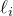, consider the parametric representation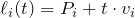. The well-known formula for the distance of two skew lines in 3D states that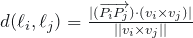.

The requirement that these distances have to be two leads to the system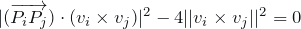,

where the squares are taken in order to avoid square roots.

Introducing coordinates for the points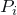and the vectors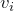and using the well-known formula for the dot product of a vector and a cross product, the authors get a system of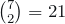polynomial equations, each of degree 6 in 12 variables.

In order to simplify the system, Bozóki et al. observe that any arrangement of seven lines can be translated and rotated to a position in which one of the lines, say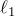, is horizontal passing through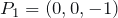in the direction of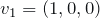. Furthermore, it can also be assumed that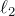goes through the point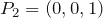, so that the touching point of the first two cylinders is the origin and the only degree of freedom for the first two cylinders is the direction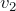of the second one.

Further choosing this direction to be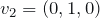(hence orthogonal to the first one), reduces the problem to finding five lines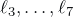, such that the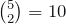distances between them, as well as their 10 distances toandare two. In this way, the system is reduced to 20 equations.

But there are still six unknowns for each line, corresponding to its point and direction vector, for a total of 30 unknowns. In order to reduce this number to 20, Bozóki et al. consider two further simplifications.

First, they observe that none of the sought five linescan be horizontal, since it would be parallel toand. Hence, for any value of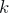all of them pass through the plane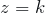. In particular, this holds for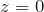, so the third coordinate of each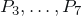can be settled to zero. This prunes out five unknowns. Second, the direction vectors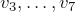are normalized so that their three coordinates sum up to one, which gets rid of five more unknowns.

In this way, Bozóki et al. come up to a system of 20 multivariate polynomial equations, of degree 6, with 20 unknowns.

The next step is showing the existence of solutions for this system. In order to do so, Bozóki et al. use symbolic and numerical computational techniques. They start by the polyhedral homotopy continuation method by Huber and Sturmfels 5, implemented in software HOM4PS-2.0 6. Using the software MixedVol-2.0 7, a total of 180,734 intermediate instances are obtained, which provide more than 120,000 million homotopy curves to be tracked.

These are processed in parallel by 12 cores in 2 Intel Xeon X5650 2.66 GHz CPUs, which is able to manage 20 million curves per month. The first solution showed up after processing 80 million curves and the second solution after another 25 million curves.

In order to verify correctness of the solutions, Bozóki et al. point up that the software HOM4PS-2.0 provides solutions up to 50 digits, of which the first 12 are ensured to be correct. This can be then used as a starting point for a floating-point arithmetic solver, like fsolve in Maple 13, which allowed the authors to check the results up to 10,000 digits.

However, since a large number of correct digits is still not mathematical correctness, they have applied two exact verifications methods: alphaCertified 8 and the interval Krawczyk method9.

This work leaves the open question of whether seven is the maximum number of mutually touching infinite cylinders. The authors note that applying the same ideas to the case of eight cylinders leads to a polynomial system of 25 variables and 27 equations, for which they are still seeking a solution.

## References

1. M. Gardner. Hexaflexagons and Other Mathematical Diversions: The First Scientific American Book of Puzzles and Games, University of Chicago Press, 1988 (reprint, first edition in 1959), pp. 110–115. ISBN: 0226282546.
2. J.E. Littlewood. Some Problems in Real and Complex Analysis, Heath Mathematical Monographs, Raytheon Education, Lexington, Massachusetts, 1968.
3. A. Bezdek. On the number of mutually touching cylinders, in: Combinatorial and Computational Geometry, in: MSRI Publication, vol. 52, 2005, pages 121-127.
4. S. Bozóki, T.-L. Lee, L. Rónyai. Seven mutually touching infinite cylinders. Computational Geometry: Theory and Applications, 48 (2015), pages 87-93. DOI: http://dx.doi.org/10.1016/j.comgeo.2014.08.007
5. B. Huber, B. Sturmfels. A polyhedral method for solving sparse polynomial systems, Mathematics of Computation, 64:212 (1995), pages 1541-1555. DOI: http://dx.doi.org/10.1090/S0025-5718-1995-1297471-4
6. T.L. Lee, T.Y. Li, C.H. Tsai. HOM4PS-2.0. A software package for solving polynomial systems by the polyhedral homotopy continuation method, Computing 83 (2008), pages 109-133. DOI: http://dx.doi.org/10.1007/s00607-008-0015-6
7. T. Chen, T.L. Lee, T.Y. Li. Mixed volume computation in parallel, Taiwanese Journal of Mathematics 18:1 (2014), pages 93-114. http://journal.taiwanmathsoc.org.tw/index.php/TJM/article/view/3276
8. J.D. Hauenstein, F. Sottile. Algorithm 921: alphaCertified: certifying solutions to polynomial systems, ACM Transactions on Mathematical Software, 38:4 (2012), article number 28. DOI: http://dx.doi.org/10.1145/2331130.2331136
9. R. Krawczyk. Newton-Algorithmen zur Bestimmung von Nullstellen mit Fehlerschranken, Computing 4:3 (1969), pages187-201. DOI: http://dx.doi.org/10.1007/BF02234767